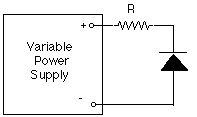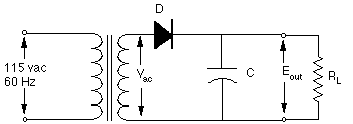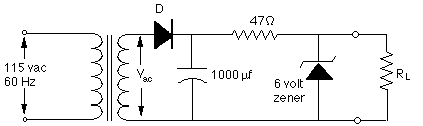Chemistry 498A/C Laboratory, Fall 1997

## Diodes and Power Supplies

Equipment required: Hitachi Model V-355 dual-trace 35 MHz oscilloscope, digital multimeter, 0-20 volt variable power supply, 6.3 volt power transformer, 10 KOhms , 10%, 1/2 watt resistor, 25 and 1000 µf, 15 volt (min) electrolytic capacitors, rectifier diode, 6 volt zener diode, selection of Si and Ge signal diodes and LEDs.

### A. Basic characteristics of diodes

1. Set up the following circuit, where the diode D is a general purpose silicon signal diode and the resistor R is a 10 KOhms , 10%, 1/2 watt resistorD

2. Set the variable power supply to several voltages between 0 and 20 volts. For each voltage, measure the voltage across the diode and the voltage across the resistor R with your digital multimeter and calculate the current through the diode from the voltage drop across R (if it's measurable).

3. Reverse the power supply polarity (or the diode polarity, which amounts to the same thing) and repeat step 2.

4. In which of these set-ups was the diode D forward biased (i.e. conducting), step 2 or step 3? ____. Reversed biased (non-conducting)? _____

5. For both the forward biased and the reverse biased case, plot the voltage across vs the current through the diode. (Attach the graphs when you turn in this report).

6. Repeat steps 2-5 for a Zener diode supplied by the instructor. What portion of the Zener diode's characteristic curve is useful for voltage regulation in dc power supplies?

### B. Simple dc power supply

1. Set up the following circuit, where the transformer is a 6-8 volt power transformer, the diode D is a rectifier diode and the resistor RL is a 1 KOhm, 1/2 watt resistor. Plug the transformer's primary winding into the 115 vac power. At first, leave out the filter capacitor C.2. Use the oscilloscope to observe the waveform across the transformer's secondary winding (i.e. Vac) and across the load resistor RL. Sketch and explain these waveforms.

3. Add a 25 µf electrolytic capacitor for the filter capacitor C. (Warning. Observe polarity. The positive end of the capacitor connects to the diode.) Observe the waveform across the load resistor RL. Explain.

4. Add a 1000 µf, 15 volt electrolytic capacitor for the filter capacitor C. (Warning. Observe polarity. The positive end of the capacitor connects to the diode.) Observe the waveform across the load resistor RL and compare to the above. Explain.

### C. Zener voltage regulator

1. Add the following Zener voltage regulator to the output of your power supply. Initially, make the load resistance RL 1000 Ohms .2.Measure the voltage across the filter capacitor ( ________ volts). Calculate the power that you expect to be dissipated by the 47 Ohms resistor (_________ watts) and by the Zener diode (_________ watts) when RL is 1000 Ohms.

3. Measure the output voltage (across RL) ________ volts. Reduce the load resistance RL to 220 Ohms and measure the output voltage across the load resistance again. ________ volts.

4. How has the use of the Zener improved the voltage regulation of this simple power supply?